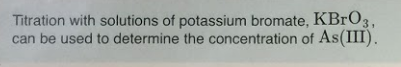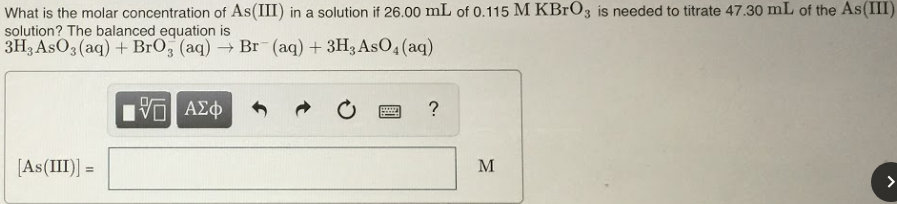# Titration with solutions of potassium bromate, KBrO3, can be used to determine the concentration of As(III). What is the molar concentration of As(III) in a solution if 26.00 mL of 0.115 MKBrO3 is needed to titrate 47.30 mL of the As(III) solution? The balanced equation is 3H3AsO3(aq) + BrO3(aq) → Br^-(aq) + 3H3AsO4(aq)